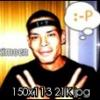# Eskimonster_DK

2

• #### Days Won

1

Eskimonster_DK had the most liked content!

1 Neutral

• Rank
Newbie

• Skype
Eskimonster

## Profile Information

• Gender
Male
• Server
EU
• Location
Århus N
• Interests
Comunities worth spending time on and games even too.

World of tanks since 10.03.2013

## Recent Profile Visitors

798 profile views
• ### Aslain

1.## Some Extra Information about Aslain's Mod Pack

Would it not be possibol to pin up the idividual mods settings options fx, Balcalmod keybinder posibillities can changed with these : "current setup is : <BalCalcKey>16</BalCalcKey> 1 = 2 2 = 3 3 = 4 4 = 5 5 = 6 6 = 7 7 = 8 8 = 9 9 = 10 0 = 11 Q = 16 W = 17 E = 18 R = 19 T = 20 Y = 21 U = 22 I = 23 O = 24 P = 25 A = 30 S = 31 D = 32 F = 33 G = 34 H = 35 J = 36 K = 37 L = 38 Z = 44 X = 45 C = 46 V = 47 B = 48 N = 49 M = 50 F1 = 59 F2 = 60 F3 = 61 F4 = 62 F5 = 63 F6 = 64 F7 = 65 F8 = 66 F9 = 67 F10 = 68 F11 = 87 F12 = 88 F13 = 100 F14 = 101 F15 = 102 MOUSE0 = 256 #ËÊÌ MOUSE1 = 257 #ÏÊÌ MOUSE2 = 258 #ÑÊÌ MOUSE3 = 259 MOUSE4 = 260 MOUSE5 = 261 MOUSE6 = 262 etc you seee my point i hope.
2. Hey from the North. First of all i would like say thanks the Creator and supporters behind this modpack and website great job there. i have always tryed to help the comunitys that came with the games i playe ,therefor i wil patron here for a while. Hope this help on your situation and contributes to the work your guys are doing. ;) In the comunity spirit :rolleyes: i would like to contribute with translation of the modinstaller into danish (DK) if it had an interest. Please pm me if so. Best regards Eskimonster_DK [DKSQN] Clan Home http://worldoftanks.eu/community/clans/500019130/ Replay Home http://wotreplays.com/uploader/Eskimonster_DK/id/383636
••